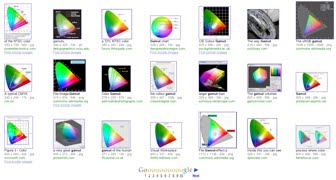## Thursday, February 18, 2010

### 1976 Uniform Chromaticity Scales: Yu'v'

A while back I came across Seyno Sluterman's short article "The NTSC Color Triangle is Obsolete, But No One Seems to Know". It's an interesting and short read but it also makes a call to use the 1976 Yu'v' Uniform Chromaticity Scales instead of the typical CIE 1931 chromaticity values when plotting display gamuts.

Which is a great reminder (1).Plotting display gamuts using CIE 1931 chromaticity values appears to be pervasive but it is obsolete.

To compute Yu'v' values, start with 1931 chromaticity of xy values and then compute u' and v' as follows:

u' = (4 * x) / ((-2 * x) + (12 * y) + 3)

v' = (9 * y) / ((-2 * x) + (12 * y) + 3)

The Y or luminance value is the same. And below, for your interactive convenience, is a simple xyY to Yu'v' calculator:

To use the above Yu'v' calculator (2), simply enter the xyY chromaticity values on the left and hit the 'Convert' button to compute the corresponding 1976 Yu'v' values. The 'Clear' button can be used to empty the Yu'v' values and reset the xyY values to 0.3333, 0.3333 and 60.

Computing Yu'v' values is nothing complicated, but it is curious that in 2010 a majority of display gamuts image search results (shown above) are plotted using a representation from 1931 that was superseded in 1976.

Footnotes
1. After my Model Based Color Tolerances paper in 1999 I had Dr. R.W.G. Hunt patiently reminded me. I glad to say I haven't needed a second reminder.
2. In this case the source JavaScript looks like:
` function computeYuvp() { document.f1.theYout.value = document.f1.theY.value; var one = parseFloat(document.f1.thex.value); var two = parseFloat(document.f1.they.value); var temp = (-2 * one) + (12 * two) + 3; document.f1.theup.value = (4 * one) / temp; document.f1.thevp.value = (9 * two) / temp; }`

1.cloud computing?

2.In the sense of abstracting details.

3.Well, maybe people see the extra computation, simple though it may be, as unnecessary. After all, WHY are people diagramming color triangles? To see, visually, how two or more compare (typically). Rarely is this comparison anything more that ordinal, i.e., "gamut A is larger than gamut B." They might even add, "in the greens". Since both diagrams answer the "which is bigger" question with equal accuracy, why use a diagram that requires more computation and may be unfamiliar (because everyone uses 1931) to many?

Certainly, it is important to remember 1976 for those times when more than an ordinal calculation is required, but for printing on datasheets...

"I came here for the color science, not math!"

4.A fair comment - certainly many color triangle diagrams are being used for as you say 'ordinal' purposes. In fact (from my experience in footnote 1 above) you can have 1931 color triangles with what appear to be minimal differences for blue primaries that are significantly larger when plotted in 1976 Yu'v' (and do indeed look significantly different). So I think even for ordinal purposes, the 4 extra multiples, 2 divides and 2 additions might be worth the effort.

We'll see about getting back to the color science now ;)